PEEI 4.0.0.1 Solver of systems of partial differential equations. Freeware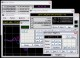Kalkulator 2.41 Advanced Sci/Eng calculator, from simple expressions to differential equations SharewareInfinity 1.3.1 Innovative non-linear math software for high precision calculations. Try it now! SharewareODE Module 1.3 ODE Module is a module that solves initial value problems of sets of coupled first-order differential equations (up to fourteen). Freeware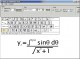Abacus Math Writer 4.0 For publishing Mathematical and Scientific equations. Shareware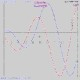ODEcalc 6.1 ODEcalc (tm): Ordinary Differential Equation (ODE) Calculator! Solves ODEs Freeware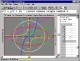Graphmatica 2.4 powerful, easy-to-use, equation plotter with numerical and calculus features Shareware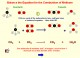Atoms, Symbols and Equations 4.0 Unique interactive multimedia Chemistry teaching software. Shareware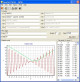EqPlot 1.3.22 EqPlot plots 2D graphs from complex equations. SharewareBerkeley Madonna 8.3.15 Berkeley Madonna is arguably the fastest, most convenient, general purpose differential equation solver available today. Shareware